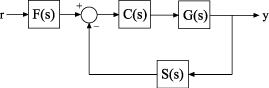# Control System Model With Both Numeric and Tunable Components

This example shows how to create a tunable model of a control system that has both fixed plant and sensor dynamics and tunable control components.

Consider the control system of the following illustration.Suppose that the plant response is $G\left(s\right)=1/\left(s+1{\right)}^{2}$, and that the model of the sensor dynamics is $S\left(s\right)=5/\left(s+4\right)$. The controller $C$ is a tunable PID controller, and the prefilter $F=a/\left(s+a\right)$ is a low-pass filter with one tunable parameter, a.

Create models representing the plant and sensor dynamics. Because the plant and sensor dynamics are fixed, represent them using numeric LTI models.

```G = zpk([],[-1,-1],1); S = tf(5,[1 4]);```

To model the tunable components, use Control Design Blocks. Create a tunable representation of the controller C.

`C = tunablePID('C','PID');`

`C` is a `tunablePID` object, which is a Control Design Block with a predefined proportional-integral-derivative (PID) structure.

Create a model of the filter $F=a/\left(s+a\right)$ with one tunable parameter.

```a = realp('a',10); F = tf(a,[1 a]);```

`a` is a `realp` (real tunable parameter) object with initial value 10. Using `a` as a coefficient in `tf` creates the tunable `genss` model object `F`.

Interconnect the models to construct a model of the complete closed-loop response from r to y.

`T = feedback(G*C,S)*F`
```T = Generalized continuous-time state-space model with 1 outputs, 1 inputs, 5 states, and the following blocks: C: Tunable PID controller, 1 occurrences. a: Scalar parameter, 2 occurrences. Type "ss(T)" to see the current value, "get(T)" to see all properties, and "T.Blocks" to interact with the blocks. ```

`T` is a `genss` model object. In contrast to an aggregate model formed by connecting only numeric LTI models, `T` keeps track of the tunable elements of the control system. The tunable elements are stored in the `Blocks` property of the `genss` model object. Examine the tunable elements of `T`.

`T.Blocks`
```ans = struct with fields: C: [1x1 tunablePID] a: [1x1 realp] ```

When you create a `genss` model of a control system that has tunable components, you can use tuning commands such as `systune` to tune the free parameters to meet design requirements you specify.